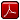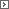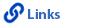Mathematics Grade 1Printable Version (pdf)Course Introduction

Core Standards of the Course

Strand: MATHEMATICAL PRACTICES (1.MP)
The Standards for Mathematical Practice in first grade describe mathematical habits of mind that teachers should seek to develop in their students. Students become mathematically proficient in engaging with mathematical content and concepts as they learn, experience, and apply these skills and attitudes (Standards 1.MP. 1�8).Standard 1.MP.1
Make sense of problems and persevere in solving them. Explain the meaning of a problem, look for entry points to begin work on the problem, and plan and choose a solution pathway. When a solution pathway does not make sense, look for another pathway that does. Explain connections between various solution strategies and representations. Upon finding a solution, look back at the problem to determine whether the solution is reasonable and accurate, often checking answers to problems using a different method or approach.Standard 1.MP.2
Reason abstractly and quantitatively. Make sense of quantities and their relationships in problem situations. Contextualize quantities and operations by using images or stories. Decontextualize a given situation and represent it symbolically. Interpret symbols as having meaning, not just as directions to carry out a procedure. Know and flexibly use different properties of operations, numbers, and geometric objects.Standard 1.MP.3
Construct viable arguments and critique the reasoning of others. Use stated assumptions, definitions, and previously established results to construct arguments. Explain and justify the mathematical reasoning underlying a strategy, solution, or conjecture by using concrete referents such as objects, drawings, diagrams, and actions. Listen to or read the arguments of others, decide whether they make sense, ask useful questions to clarify or improve the arguments, and build on those arguments.Standard 1.MP.4
Model with mathematics. Identify the mathematical elements of a situation and create a mathematical model that shows the relationships among them. Identify important quantities in a contextual situation, use mathematical models to show the relationships of those quantities, analyze the relationships, and draw conclusions. Models may be verbal, contextual, visual, symbolic, or physical.Standard 1.MP.5
Use appropriate tools strategically. Consider the tools that are available when solving a mathematical problem, whether in a real-world or mathematical context. Choose tools that are relevant and useful to the problem at hand, such as physical objects, drawings, diagrams, physical tools, technologies, or mathematical tools, such as estimation or a particular strategy or algorithm.

Standard 1.MP.6
Attend to precision. Communicate precisely to others by crafting careful explanations that communicate mathematical reasoning by referring specifically to each important mathematical element, describing the relationships among them, and connecting their words clearly to representations. Calculate accurately and efficiently, and use clear and concise notation to record work.

Standard 1.MP.7
Look for and make use of structure. Recognize and apply the structures of mathematics such as patterns, place value, the properties of operations, or the flexibility of numbers. See complicated things as single objects or as being composed of several objects.

Standard 1.MP.8
Look for and express regularity in repeated reasoning. Notice repetitions in mathematics when solving multiple related problems. Use observations and reasoning to find shortcuts or generalizations. Evaluate the reasonableness of intermediate results.

Strand: OPERATIONS AND ALGEBRAIC THINKING (1.OA)
Represent and solve problems involving addition and subtraction within 20 (Standards 1�2, 5�6). Understand and apply properties of operations and the relationship between addition and subtraction (Standards 3�4). Work with addition and subtraction equations (Standards 7�8).Standard 1.OA.1
Use addition and subtraction within 20 to solve word problems involving situations of adding to, taking from, putting together, taking apart, and comparing, with unknowns in all positions. For example, use objects, drawings, and equations with a symbol for the unknown number to represent the problem.Standard 1.OA.2
Solve word problems that call for addition of three whole numbers whose sum is less than or equal to 20. For example, use objects, drawings, and equations with a symbol for the unknown number to represent the problem.Standard 1.OA.3
Apply properties of operations as strategies to add and subtract. For example: If 8 + 3 = 11 is known, then 3 + 8 = 11 is also known. (Commutative property of addition.) To add 2 + 6 + 4, the second two numbers can be added to make a ten, so 2 + 6 + 4 = 2 + 10 =12. (Associative property of addition.) First grade students need not use formal terms for these properties.Standard 1.OA.4
Understand subtraction as an unknown-addend problem. For example, subtract 10 � 8 by finding the number that makes 10 when added to 8.Standard 1.OA.5
Relate counting to addition and subtraction. For example, by counting on 2 to add 2.Standard 1.OA.61. Use strategies such as counting on; making ten (for example, 8 + 6 = 8 + 2 + 4 = 10 + 4 = 14); decomposing a number leading to a ten (for example, 13 - 4 = 13 � 3 � 1 = 10 � 1 = 9); using the relationship between addition and subtraction (for example, knowing that 8 + 4 = 12, one knows 12 � 8 = 4); and creating equivalent but easier or known sums (for example, adding 6 + 7 by creating the known equivalent 6 + 6 + 1 = 12 + 1 = 13).
2. By the end of Grade 1, demonstrate fluency for addition and subtraction within 10.

Standard 1.OA.7
Understand the meaning of the equal sign, and determine whether equations involving addition and subtraction are true or false. For example, which of the following equations are true and which are false? 6 = 6, 7 = 8 � 1, 5 + 2 = 2 + 5, 4 + 1 = 5 + 2.Standard 1.OA.8
Determine the unknown whole number in an addition or subtraction equation relating three whole numbers. For example, determine the unknown number that makes the equation true in each of the equations 8 + ? = 11, 5 = ? � 3, 6 + 6 = ?Strand: NUMBER AND OPERATIONS IN BASE TEN (1.NBT)
Extend the counting sequence (Standard 1). Understand place value (Standards 2�3). Use place value understanding and properties of operations to add and subtract (Standards 4�6).Standard 1.NBT.1
Count to 120, starting at any number less than 120. In this range, read and write numerals and represent a number of objects with a written numeral.Standard 1.NBT.2
Understand that the two digits of a two-digit number represent amounts of tens and ones. Understand the following as special cases:1. 10 can be thought of as a bundle of ten ones, called a "ten."
2. The numbers from 11 to 19 are composed of a ten and one, two, three, four, five, six, seven, eight, or nine ones.
3. The numbers 10, 20, 30, 40, 50, 60, 70, 80, 90 refer to one, two, three, four, five, six, seven, eight, or nine tens (and 0 ones).

Standard 1.NBT.3
Compare two two-digit numbers based on meanings of the tens and ones digits, recording the results of comparisons with the symbols >, =, and <.Standard 1.NBT.4
Add within 100, including adding a two-digit number and a one-digit number, and adding a two-digit number and a multiple of 10, using concrete models or drawings and strategies based on place value, properties of operations, and/or the relationship between addition and subtraction; relate the strategy to a written method and explain the reasoning used. Understand that in adding two-digit numbers, one adds tens to tens and ones to ones, and that it is sometimes necessary to compose a ten.Standard 1.NBT.5
Given a two-digit number, mentally find 10 more or 10 less than the number, without having to count; explain the reasoning used.Standard 1.NBT.6
Subtract multiples of 10 in the range 10�90 from multiples of 10 in the range 10�90 (positive or zero differences), using concrete models or drawings and strategies based on place value, properties of operations, and/or the relationship between addition and subtraction; relate the strategy to a written method and explain the reasoning used.Strand: MEASUREMENT AND DATA (1.MD.)
Measure lengths indirectly and by iterating length units (Standards 1�2). Tell and write time (Standard 3). Represent and interpret data (Standard 4). Identify the value of coins (Standard 5).Standard 1.MD.1
Order three objects by length; compare the lengths of two objects indirectly by using a third object.Standard 1.MD.2
Express the length of an object as a whole number of length units, by laying multiple copies of a shorter object (the length unit) end to end; understand that the length measurement of an object is the number of same-size length units that span it with no gaps or overlaps. Limit to contexts where the object being measured is spanned by a whole number of length units with no gaps or overlaps.Standard 1.MD.3
Tell and write time in hours and half-hours using analog and digital clocks.Standard 1.MD.4
Organize, represent, and interpret data with up to three categories; ask and answer questions about the total number of data points, how many in each category, and how many more or less are in one category than in another.Standard 1.MD.5
Identify the values of pennies, nickels, dimes and quarters and know their comparative values. (For example, a dime is of greater value than a nickel.) Use appropriate notation to designate a coin�s value. (For example, 5¢.)Strand: GEOMETRY (1.G.)
Reason with shapes and their attributes (Standards 1�3).Standard 1.G.1
Distinguish between defining attributes (for example, triangles are closed and three-sided) versus non-defining attributes (for example, color, orientation, overall size); build and draw shapes that possess defining attributes.Standard 1.G.2
Compose shapes.1. Compose two-dimensional shapes (rectangles, squares, trapezoids, triangles, halfcircles, and quarter-circles) to create a composite shape, and compose new shapes from the composite shape.
2. Compose three-dimensional shapes (cubes, right rectangular prisms, right circular cones, and right circular cylinders) to create a composite shape, and compose new shapes from the composite shape. First grade students do not need to learn formal names such as "right rectangular prism."

Standard 1.G.3
Partition circles and rectangles into two and four equal shares; describe the shares using the words halves, fourths, and quarters; and use the phrases half of, fourth of, and quarter of. Describe the whole as two or four of the shares. Understand that, for these examples, decomposing into more equal shares creates smaller shares.http://www.uen.org - in partnership with Utah State Board of Education (USBE) and Utah System of Higher Education (USHE).  Send questions or comments to USBE Specialist - Shannon Olson and see the Mathematics - Elementary website. For general questions about Utah's Core Standards contact the Director - Jennifer Throndsen .

These materials have been produced by and for the teachers of the State of Utah. Copies of these materials may be freely reproduced for teacher and classroom use. When distributing these materials, credit should be given to Utah State Board of Education. These materials may not be published, in whole or part, or in any other format, without the written permission of the Utah State Board of Education, 250 East 500 South, PO Box 144200, Salt Lake City, Utah 84114-4200.